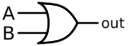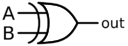# Difference between revisions of "Digital Electronics"

This topic is an extension of the topic of Boolean Algebra which includes a more thorough description of the category in terms of determining whether a circuit results in a TRUE or FALSE value using truth tables or how to simplify a circuit to as few gates as possible. Electrical engineers use the following symbols to design electrical circuits that are used inside the computer’s hardware. The following table illustrates the equivalent Boolean algebra expression and truth table for each gate.

# Definitions

NAME GRAPHICAL SYMBOL ALGEBRAIC EXPRESSION TRUTH TABLE
BUFFER Symbol X = A
A X
0 0
1 1
NOT Symbol X = $\overline{A}$
A X INPUT OUPUT 1 0
ANDX = $AB$ or $A \cdot B$
A B X INPUT OUPUT 0 0 0 0 1 0 1 0 0 1 1 1
NAND Symbol X = $\overline{AB}$ or $\overline{A\cdot B}$
A B X
0 0 1
0 1 1
1 0 1
1 1 0
ORX = $A+B$
A B X
0 0 0
0 1 1
1 0 1
1 1 1
NOR Symbol X = $\overline{A+B}$
A B X
0 0 1
0 1 0
1 0 0
1 1 0
XORX = $A \oplus B$
A B X
0 0 0
0 1 1
1 0 1
1 1 0
XNOR Symbol X = $\overline{A \oplus B} \text{ or } A \odot B$
A B X
0 0 1
0 1 0
1 0 0
1 1 1

# Sample Problems

## Sample Problem 1

Find all ordered 4-tuples (A, B, C, D), which make the following circuit FALSE:

Solution: This circuit diagram translates to: $(\overline{C+D}+B) \oplus (\overline{A}B) \oplus (\overline{C+D})$. The table has the following headings: 1=$\overline{C+D}$, 2=$1+\overline{B}$, 3=$\overline{A}B$, 4=$A \oplus B$, and 5=$4 \oplus 1$. Thus, the 4-tuples (0,0,0,0), (1,0,0,0), (1,1,0,0), (1,1,0,1), (1,1,1,0), and (1,1,1,1) all make the circuit FALSE.

$A$ $B$ $C$ $D$ $1$ $2$ $3$ $4$ $5$
0 0 0 0 1 1 0 1 0
0 0 0 1 0 1 0 1 1
0 0 1 0 0 1 0 1 1
0 0 1 1 0 1 0 1 1
0 1 0 0 1 1 1 0 1
0 1 0 1 0 0 1 1 1
0 1 1 0 0 1 1 1 1
0 1 1 1 0 0 1 1 1
1 0 0 0 1 1 0 1 0
1 0 0 1 0 1 0 1 1
1 0 1 0 0 1 0 1 1
1 0 1 1 0 1 0 1 1
1 1 0 0 1 1 0 1 0
1 1 0 1 0 0 0 0 0
1 1 1 0 0 0 0 0 0
1 1 1 1 0 0 0 0 0

## Sample Problem 2

Find all ordered triplets (A, B, C) which make the following circuit FALSE:

Solution: The circuit translates to the Boolean expression $\overline{AB}+C$. To find when this is FALSE we can equivalently find when the $\overline{\overline{AB}+C}$ is TRUE. We can simplify this by applying DeMorgan’s Law and cancelling the double not over AB to yield $AB\overline{C}$ . This is TRUE when for (1,1,0) only.

## Sample Problem 3

Simplify the following digital circuit as a Boolean algebra expression using the fewest gates possible:

PICTURE GOES HERE

Solution: This translates to: $\overline{A\cdot B+\overline{C}\cdot D}$ . Simplify: $\overline{(A\cdot B+\overline{C})\cdot D}=\overline{AB+\overline{C}}+\overline{D}$ $=\overline{AB}\cdot \overline{\overline{C}}+\overline{D}=(\overline{A}+B)C+\overline{D}$ This has 5 gates and $\overline{A}C+BC+\overline{D}$ has 6 so the above expression is best.

# Video Resources

The following YouTube videos show ACSL students and advisors working out some ACSL problems that have appeared in previous contests. Some of the videos contain ads; ACSL is not responsible for the ads and does not receive compensation in any form for those ads.